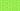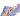PricingResources

# What is financial ratio analysis?

Written by

Financial ratio analysis is the technique of comparing the relationship (or ratio) between two or more items of financial data from a company’s financial statements. It is mainly used as a way of making fair comparisons across time and between different companies or industries.

## Financial ratio analysis and interpretation

Financial ratio analysis is generally used in six main areas. These are:

• liquidity

• coverage

• solvency

• profitability

• efficiency

• market prospects

It’s important to keep in mind that financial ratios can only be interpreted fairly if the financial statements on which they are based are prepared according to the same set of accounting standards. This ensures that the underlying general ledger accounts always relate to the same line items in the financial statements.

## An overview of key financial ratio analysis

Here is a quick guide to the main types of financial ratio analysis and the key financial ratios used in them.

### Liquidity

Liquidity analysis is used to analyse a company’s abilities to meet its immediate debt obligations out of its current assets. The two key financial ratios used to analyse liquidity are:

• Current ratio = current assets divided by current liabilities

• Quick ratio = (current assets minus inventory) divided by current liabilities

The current ratio is also known as the working capital ratio and the quick ratio is also known as the acid test ratio.

### Coverage

Coverage analysis is used to analyse a company’s ability to pay interest, fees and charges on its debts but not the underlying capital obligations. The two key financial ratios used to analyse solvency are:

• Times-interest-earned ratio = earnings before interest and taxes divided by interest expense

• Debt-service-coverage ratio = net operating income divided by total debt service charges

### Solvency

Solvency analysis is used to analyse a company’s ability to pay off all the debt it currently holds with its income, assets and divided by or equity. The two key financial ratios used to analyse solvency are:

• Total -debt ratio = total liabilities divided by total assets

• Debt-to-equity ratio = total liabilities divided by (total assets minus total liabilities)

### Profitability

Profitability analysis is used to analyse a company’s ability to make money from its goods and divided by or services. The four key financial ratios used to analyse profitability are:

• Net profit margin = net income divided by sales

• Return on total assets = net income divided by assets

• Basic earning power = EBIT divided by total assets

• Return on equity = net income divided by common equity

### Efficiency

Efficiency analysis is used to analyse how hard a business is working its assets on behalf of its owners. The four key financial ratios used to analyse efficiency are:

• Inventory-turnover ratio = sales divided by inventory

• Days-sales outstanding = accounts receivable divided by average sales per day

• Fixed-assets-turnover ratio = sales divided by net fixed assets

• Total-assets-turnover ratio = sales divided by total assets

Day-sales outstanding is also known as the average collection period.

### Market prospects

Market prospects analysis is generally only undertaken for publicly traded companies. It is generally used to determine the likely prospects of different investment options.

There are numerous financial ratios used to calculate market prospects. Key ones include:

• Price-earnings ratio = stock price per share divided by earnings per share

• Price-cash-flow ratio = stock price divided by cash flow per share

• Market-book ratio = stock price divided by book value per share

• Dividend yield = dividend divided by share price

• Earnings-per-share = profit divided by number of outstanding shares

• Dividend-payout ratio = dividend per share divided by earnings per share or dividends divided by net income

## Limitations of financial ratio analysis

Financial ratio analysis is quantitative rather than qualitative. It, therefore, does not address certain factors which can play a huge role in determining a company’s prospects. For example, it cannot analyse the quality of their management. This means that, although financial ratio analysis can be hugely useful, it only tells part of the story.

## We can help

Financial ratio analysis can be applied in lots of other contexts too.  For example, the accounts receivable days formula can help you to understand whether or not an accounts-receivable process is working efficiently.## Interested in automating the way you get paid? GoCardless can help

Contact sales

Sales

Contact Sales

+1(415) 523-2279

Support

help@gocardless.com

+1 (628) 241-0044